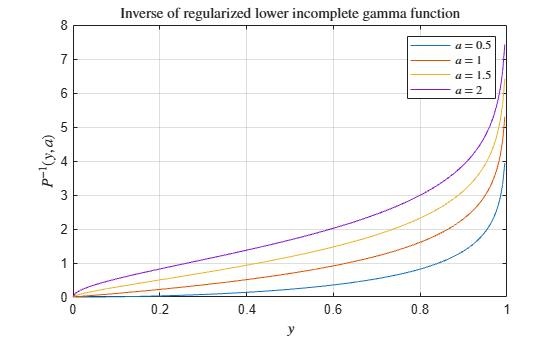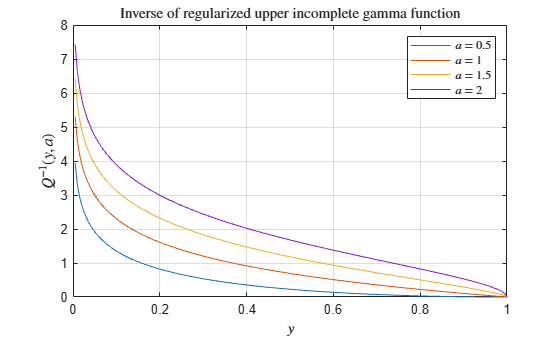# gammaincinv

Inverse of regularized incomplete gamma function

## Syntax

``X = gammaincinv(Y,A)``
``X = gammaincinv(Y,A,type)``

## Description

example

````X = gammaincinv(Y,A)` returns the inverse of the regularized lower incomplete gamma function evaluated at the elements of `Y` and `A`, such that `Y = gammainc(X,A)`. Both `Y` and `A` must be real. The elements of `Y` must be in the closed interval `[0,1]` and `A` must be nonnegative.```

example

````X = gammaincinv(Y,A,type)` returns the inverse of the regularized lower or upper incomplete gamma function. The choices for `type` are `'lower'` (the default) and `'upper'`.```

## Examples

collapse all

Calculate the inverse of the regularized lower incomplete gamma function for $a$ = 0.5, 1, 1.5, and 2 within the interval $0\le y\le 1$. Loop over values of $a$, evaluate the inverse function at each one, and assign each result to a column of `X`.

```A = [0.5 1 1.5 2]; Y = 0:0.005:1; X = zeros(201,4); for i = 1:4 X(:,i) = gammaincinv(Y,A(i)); end```

Plot all of the inverse functions in the same figure.

```plot(Y,X) grid on legend('\$a = 0.5\$','\$a = 1\$','\$a = 1.5\$','\$a = 2\$','interpreter','latex') title('Inverse of regularized lower incomplete gamma function','interpreter','latex') xlabel('\$y\$','interpreter','latex') ylabel('\$P^{-1}(y,a)\$','interpreter','latex')```Calculate the inverse of the regularized upper incomplete gamma function for $a$ = 0.5, 1, 1.5, and 2 within the interval $0\le y\le 1$. Loop over values of $a$, evaluate the inverse function at each one, and assign each result to a column of `X`.

```A = [0.5 1 1.5 2]; Y = 0:0.005:1; X = zeros(201,4); for i = 1:4 X(:,i) = gammaincinv(Y,A(i),'upper'); end```

Plot all of the inverse functions in the same figure.

```plot(Y,X) grid on legend('\$a = 0.5\$','\$a = 1\$','\$a = 1.5\$','\$a = 2\$','interpreter','latex') title('Inverse of regularized upper incomplete gamma function','interpreter','latex') xlabel('\$y\$','interpreter','latex') ylabel('\$Q^{-1}(y,a)\$','Interpreter','latex')```## Input Arguments

collapse all

Input array, specified as a scalar, vector, matrix, or multidimensional array. The elements of `Y` must be real and within the closed interval `[0,1]`. `Y` and `A` must be the same size, or else one of them must be a scalar.

Data Types: `single` | `double`

Input array, specified as a scalar, vector, matrix, or multidimensional array. The elements of `A` must be real and nonnegative. `Y` and `A` must be the same size, or else one of them must be a scalar.

Data Types: `single` | `double`

Type of inverse incomplete gamma function, specified as `'lower'` or `'upper'`. If `type` is `'lower'`, then `gammainc` returns the inverse of the regularized lower incomplete gamma function. If `type` is `'upper'`, then `gammainc` returns the inverse of the regularized upper incomplete gamma function.

collapse all

### Inverse of Incomplete Gamma Function

The inverse of the regularized lower incomplete gamma function is defined as $x={P}^{-1}\left(y,a\right)$, such that

`$y=P\left(x,a\right)=\frac{1}{\Gamma \left(a\right)}{\int }_{0}^{x}{t}^{a-1}{e}^{-t}dt.$`

The inverse of the regularized upper incomplete gamma function is defined as $x={Q}^{-1}\left(y,a\right)$, such that

`$y=Q\left(x,a\right)=\frac{1}{\Gamma \left(a\right)}{\int }_{x}^{\infty }{t}^{a-1}{e}^{-t}dt.$`

The $\Gamma \left(a\right)$ term is the gamma function

`$\Gamma \left(a\right)={\int }_{0}^{\infty }{t}^{a-1}{e}^{-t}dt.$`

MATLAB® uses the regularized or normalized definition of the incomplete gamma function, where $P\left(x,a\right)+Q\left(x,a\right)=1$.

Some properties of the inverse of the regularized lower incomplete gamma function are:

• $\underset{y\to 1}{\mathrm{lim}}{P}^{-1}\left(y,a\right)=\infty \text{ }\text{for}\text{\hspace{0.17em}}a>0$

• $\underset{\begin{array}{l}y\to 1\\ a\to 0\end{array}}{\mathrm{lim}}{P}^{-1}\left(y,a\right)=0$

## Tips

• When the regularized upper incomplete gamma function is close to 0, specifying the `'upper'` option to calculate the upper inverse function is more accurate than subtracting the regularized lower incomplete gamma function from 1 and then taking the lower inverse function.

 Olver, F. W. J., A. B. Olde Daalhuis, D. W. Lozier, B. I. Schneider, R. F. Boisvert, C. W. Clark, B. R. Miller, and B. V. Saunders, eds., Chapter 8. Incomplete Gamma and Related Functions, NIST Digital Library of Mathematical Functions, Release 1.0.22, Mar. 15, 2018.